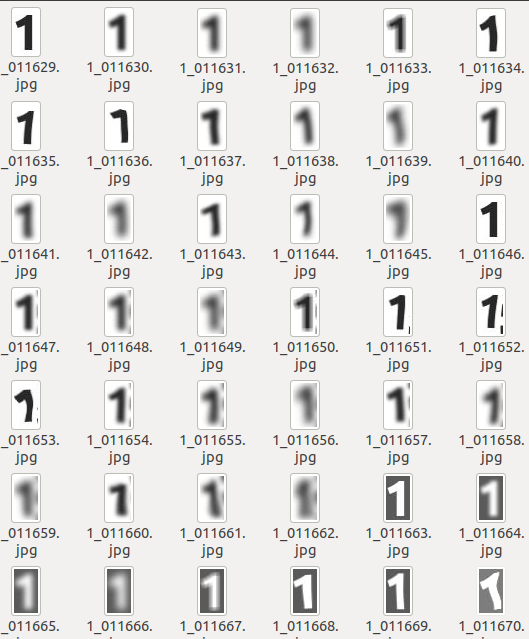Instantly share code, notes, and snippets.

#erniejunior/elastic_transform.py forked from fmder/elastic_transform.py Last active Dec 9, 2019

Elastic transformation of an image in Python
 import numpy as np from scipy.ndimage.interpolation import map_coordinates from scipy.ndimage.filters import gaussian_filter def elastic_transform(image, alpha, sigma, random_state=None): """Elastic deformation of images as described in [Simard2003]_. .. [Simard2003] Simard, Steinkraus and Platt, "Best Practices for Convolutional Neural Networks applied to Visual Document Analysis", in Proc. of the International Conference on Document Analysis and Recognition, 2003. """ if random_state is None: random_state = np.random.RandomState(None) shape = image.shape dx = gaussian_filter((random_state.rand(*shape) * 2 - 1), sigma, mode="constant", cval=0) * alpha dy = gaussian_filter((random_state.rand(*shape) * 2 - 1), sigma, mode="constant", cval=0) * alpha dz = np.zeros_like(dx) x, y, z = np.meshgrid(np.arange(shape), np.arange(shape), np.arange(shape)) print x.shape indices = np.reshape(y+dy, (-1, 1)), np.reshape(x+dx, (-1, 1)), np.reshape(z, (-1, 1)) distored_image = map_coordinates(image, indices, order=1, mode='reflect') return distored_image.reshape(image.shape)
Owner Author

### erniejunior commented Jan 11, 2016

 This version also supports color images (3 RGB channels).

### jdelange commented Sep 2, 2016

 Nice! What are good values for alpha and sigma? I assume the alpha from the original paper (a=8) cannot be directly translated to this implementation?

### mamrehn commented Nov 24, 2016

 Thanks! A note: `dz` defined in line L18 is actually never used (in L22). For RGB images it makes sense not to mix channels. In that case, you can just delete the line with `dz = np.zeros_like(dx)`.

### lgy1425 commented Apr 17, 2017

 Thank you. But should Input Image be square?

### iliya-hajjar commented Nov 19, 2017

 How can I save this distored image ? I tried with PIL and scipy but the output is entirely black , actually nothing. I can't even show the image with matplot. (TypeError: Invalid dimensions for image data) The error is clear but how can I create the appropriate shape for images?

### l770943527 commented Feb 26, 2018 • edited

 Hi, I have load a RGB img whose shape is (400, 248, 3), but I have got an error `ValueError: operands could not be broadcast together with shapes (248,400,3) (400,248,3) ` in the code here `indices = np.reshape(y + dy, (-1, 1)), np.reshape(x + dx, (-1, 1)), np.reshape(z, (-1, 1)) ` can anyone help ? THX!!!

### iver56 commented Oct 15, 2018 • edited

 Nice! What are good values for alpha and sigma?

### bigfred76 commented Jan 17, 2019

 Hi, I have load a RGB img whose shape is (400, 248, 3), but I have got an error `ValueError: operands could not be broadcast together with shapes (248,400,3) (400,248,3) ` in the code here `indices = np.reshape(y + dy, (-1, 1)), np.reshape(x + dx, (-1, 1)), np.reshape(z, (-1, 1)) ` can anyone help ? THX!!! you need to invert the shapes in the resolution of x,y,z : x, y, z = np.meshgrid(np.arange(shape), np.arange(shape), np.arange(shape)) instead of x, y, z = np.meshgrid(np.arange(shape), np.arange(shape), np.arange(shape))

### bigfred76 commented Jan 17, 2019 • edited

 with the correction I gave in the previous post, the algo works perfectly ! Thanks Results on the dataset of OCR digits recognition I'm currently building :For the question of sigma alpha values, I build the dataset with 3 pairs of values as follows : ELASTIC_ALPHA_SIGMA = ((1201, 10), (1501, 12), (991, 8))

### Rsalganik1123 commented Mar 4, 2019

 Hello I get an error: tuple index out of range on line : x, y, z = np.meshgrid(np.arange(shape), np.arange(shape), np.arange(shape)) Anyone have any advice?

### noorulhasan06 commented Apr 13, 2019

 try to print the shape and you will find out that the shape is something like [x,y] not [x,y,z]. this can be because you may using grayscale image. try to reshape the image to [x,y,1].